HomeBlogData Science

# 21 Top Data Scientist Interview Questions

Explore the top data science interview questions with answers for final-year students and professionals looking for jobs.
Dec 2022  · 21 min readIn this post, we have outlined the most frequently asked questions during the statistical and machine learning, analysis, coding, and product-sense interview stages. Practicing these data scientist interview questions will help students looking for internships and professionals looking for jobs clear all of the technical interview stages.

## General Data Science Interview Questions

### What are the assumptions required for a Linear Regression?

The four assumptions from a linear regression are:

1. Linear Relationship: there should be a linear relationship between independent variable x and dependent variable y.
2. Independence: there is no correlation between consecutive residuals. It mostly occurs in time-series data.
3. Homoscedasticity: at every level of x, the variance should be constant.
4. Normality: the residuals are normally distributed.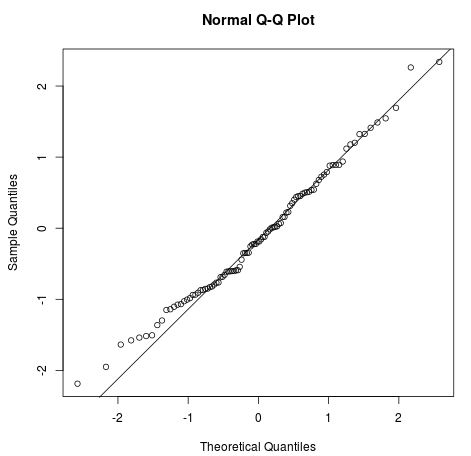Image from Statology

You can explore the concepts and applications of linear models by taking our Introduction to Linear Modeling in Python course.

### How do you handle a dataset missing several values?

There are various ways to handle missing data. You can:

1. Drop the rows with missing values.
2. Drop the columns with several missing values.
3. Fill the missing value with a string or numerical constant.
4. Replace the missing values with the average or median value of the column.
5. Use multiple-regression analyses to estimate a missing value.
6. Use multiple columns to replace missing values with average simulated values and random errors.

Learn how to diagnose, visualize and treat missing data by completing Handling Missing Data with Imputations in R course.

### How do you explain technical aspects of your results to stakeholders with a non-technical background?

First, you need to learn more about the stakeholder’s background and use this information to modify your wording. If he has a finance background, learn about commonly used terms in finance and use them to explain the complex methodology.

Second, you need to use a lot of visuals and graphs. People are visual learners, as they learn immensely better with the use of creative communication tools.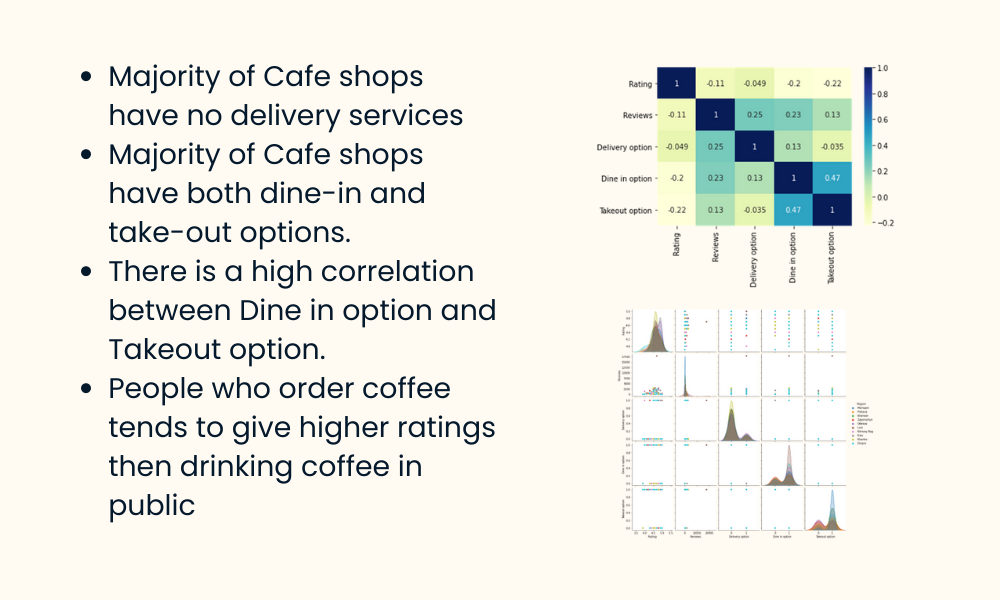Image by Author

Third, speak in terms of results. Don’t try to explain the methodologies or statistics. Try to focus on how they can use the information from the analysis to improve the business or workflow.

Finally, encourage them to ask you questions. People are scared or even embarrassed to ask questions on unknown subjects. Create a two-way communication channel by engaging them in the discussion.

Learn to build your own SQL reports and dashboards by taking our Reporting in SQL course.

## Data Science Technical Interview Questions

### What are the feature selection methods used to select the right variables?

There are three main methods for feature selection: filter, wrapper, and embedded methods.

Filter Methods

Filter methods are generally used in preprocessing steps. These methods select features from a dataset independent of any machine learning algorithms. They are fast, require fewer resources, and remove duplicated, correlated, and redundant features.Image by Author

Some techniques used are:

• Variance Threshold
• Correlation Coefficient
• Chi-Square test
• Mutual Dependence

Wrapper Methods

In wrapper methods, we train the model iteratively using a subset of features. Based on the results of the trained model, more features are added or removed. They are computationally more expensive than filter methods but provide better model accuracy.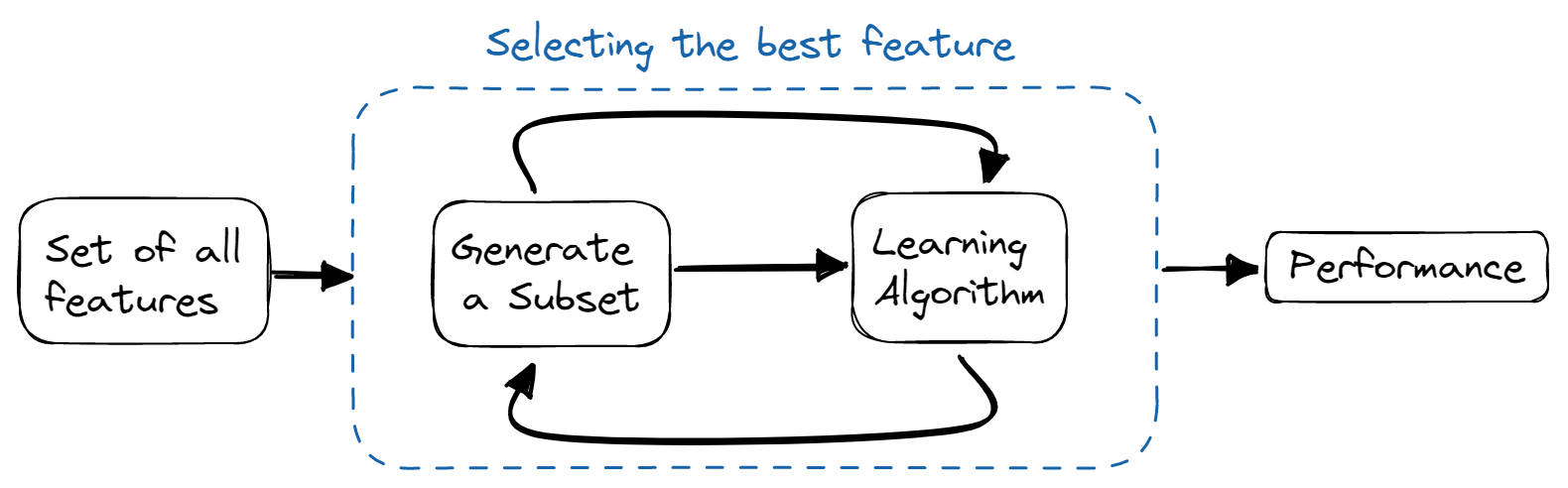Image by Author

Some techniques used are:

• Forward selection
• Backward elimination
• Bi-directional elimination
• Recursive elimination

Embedded Methods

Embedded methods combine the qualities of filter and wrapper methods. The feature selection algorithm is blended as a part of the learning algorithm, providing the model with a built-in feature selection method. These methods are faster, like the filter methods, accurate like the wrapper methods, and take into consideration a combination of features as well.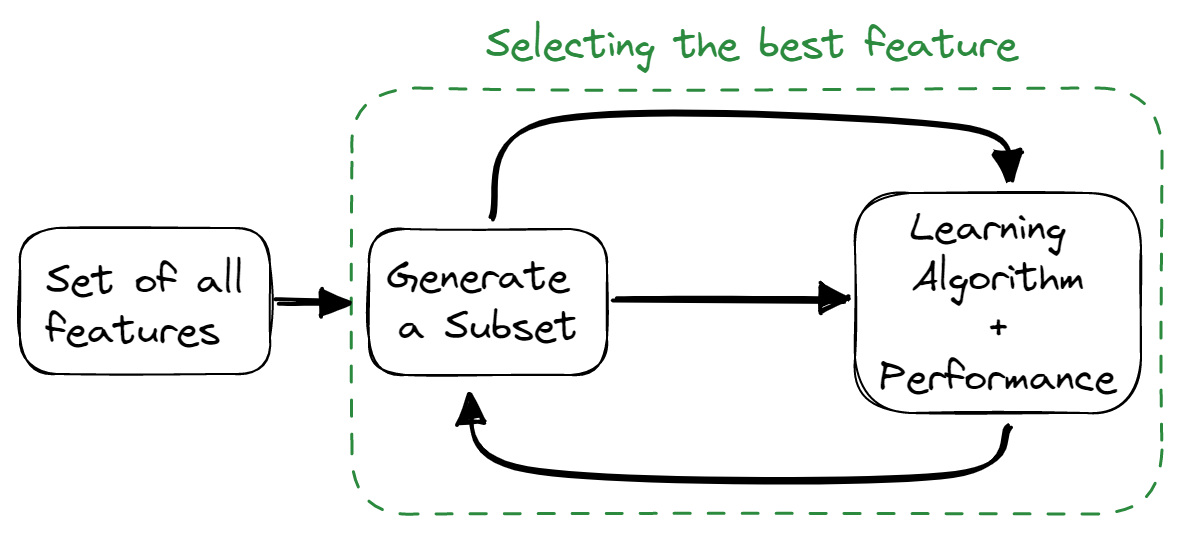Image by Author

Some techniques used are:

• Regularization
• Tree-based methods

### How can you avoid overfitting your model?

Overfitting refers to a model that is trained too well on a training dataset but fails on the test and validation dataset.

You can avoid overfitting by:

1. Keeping the model simple by decreasing the model complexity, taking fewer variables into account, and reducing the number of parameters in neural networks.
2. Using cross-validation techniques.
3. Training the model with more data.
4. Using data augmentation that increases the number of samples.
5. Using ensembling (Bagging and Boosting)
6. Using regularization techniques to penalize certain model parameters if they're likely to cause overfitting.

### List the different types of relationships in SQL

There are four main types of SQL relationships:

• One to One: it exists when each record of a table is related to only one record in another table.
• One to Many and Many to One:  it is the most common connection, where each record in a table is linked to several records in another.
• Many to Many: it exists when each record of the first table is related to more than one record in the second table, and a single record in the second table can be related to more than one record of the first table.
• Self-Referencing Relationships: it occurs when a table has to declare a connection with itself.

Learn how to explore the tables, the relationships between them, and the data stored in them by completing our Exploratory Data Analysis in SQL course.

### What are dimensionality reduction and its benefits?

Dimensionality reduction is a process that converts the dataset from several dimensions into fewer dimensions while maintaining similar information.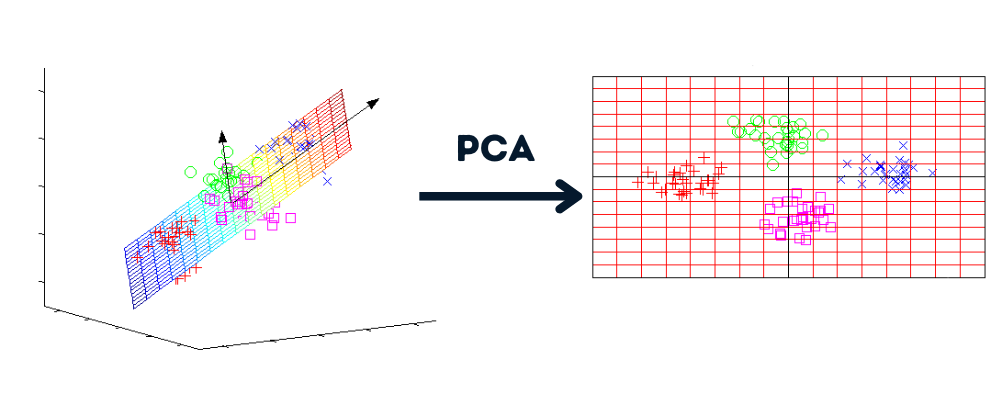Image by Author | Graphs by howecoresearch

Benefits of dimensionality reduction:

1. Compressing the data and reducing the storage space.
2. Reduce computational time and allow us to perform faster data processing.
3. It removes redundant features, if any.

Understand the concept of reducing dimensionality and master the techniques by practicing using Dimensionality Reduction in Python course.

### What is the goal of A/B Testing?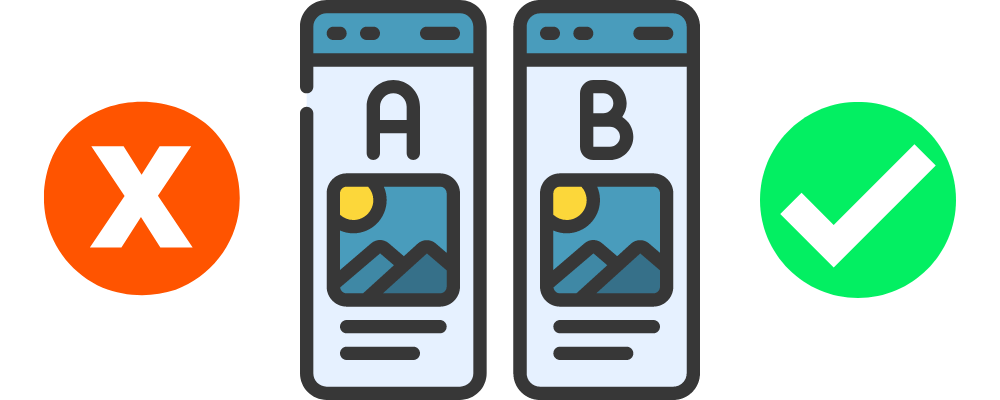Image by Author

A/B testing eliminates the guesswork and helps us make data-driven decisions to optimize the product or website. It is also known as split testing, where randomized experiments are conducted to analyze two or more versions of variables (web page, app feature, etc.) and determine which version drives maximum traffic and business metric.

Learn how to create, run, and analyze A/B tests by taking our Customer Analytics and A/B Testing in Python course.

## Data Science Coding Interview Questions

### Given a dictionary consisting of many roots and a sentence, stem all the words in the sentence with the root forming it.

Stemming is commonly used in text and sentiment analysis. In this question, you will write a Python function that will convert certain words from the list to their root form - Interview Query.

Input:

The function will take two arguments: list of root words and sentence.

``````roots = ["cat", "bat", "rat"]
sentence = "the cattle was rattled by the battery"``````

Output:

It will return the sentence with root words.

``"the cat was rat by the bat"``

Before you jump into writing code, you need to understand that we will perform two tasks: check if the word has a root and replace it.

1. You will split the sentence into words.
2. Run the outer loop over each word in the list and the inner loop over the list of root words.
3. Check if the word starts with the root. The Python string provides us with the `startswith()` function to perform this task.
4. If the word starts with the root, replace the word with the root using a list index.
5. Join all of the words to create a sentence.
``````roots = ["cat", "bat", "rat"]
sentence = "the cattle was rattled by the battery"

def replace_words(roots, sentence):
words = sentence.split(" ")

# looping over each word
for index, word in enumerate(words):
# looping over each root
for root in roots:
if word.startswith(root):
# replacing the word with its root
words[index] = root
return " ".join(words)

replace_words(roots, sentence)

# 'the cat was rat by the bat'``````

### Check if String is a Palindrome

Given the string `text`, return `True` if it’s a palindrome, else `False`.

After lowering all letters and removing all non-alphanumeric characters, the word should read the same forward and backward.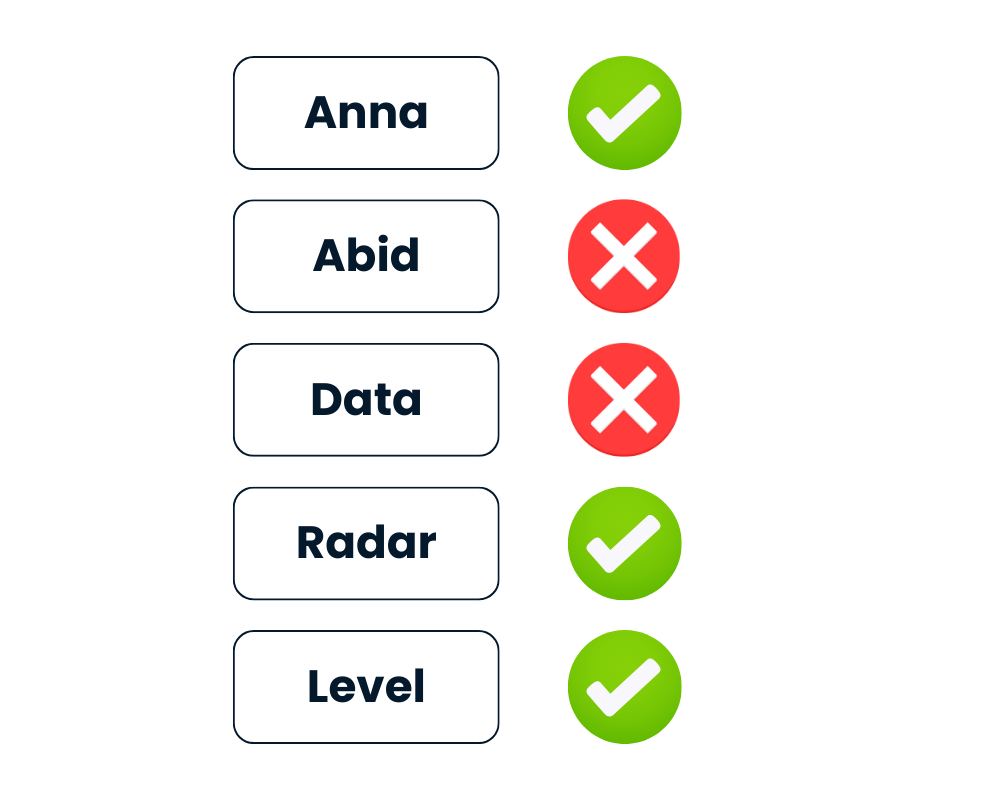Image by Author

Python provides easy ways to solve this challenge. You can either treat the string as iterable and reverse it using `text[::-1]` or use the built-in `reversed(text)` method.

1. First, you will lower the text.
2. Clean the text by removing non-alphanumeric characters using regex.
3. Reverse the text using `[::-1]`.
4. Compare the cleaned text with the reversed text.
``````import re

def is_palindrome(text):
# lowering the string
text = text.lower()

# Cleaning the string
rx = re.compile('\W+')
text = rx.sub('',text).strip()

# Reversing and comparing the string
return text == text[::-1]``````

In the second method, you will just replace reversing the text with `''.join(reversed(text))` and compare it with cleaned text.

Both methods are straightforward.

``````def is_palindrome(text):
# lowering the string
text = text.lower()

# Cleaning the string
rx = re.compile('\W+')
text = rx.sub('',text).strip()

# Reversing the string
rev = ''.join(reversed(text))
return text == rev``````

Results:

We will provide the list of the word to the `is_palindrome()` function and print the results. As you can see, even with special characters, the function has identified “Level” and “Radar” as palindromes.

``````# Test cases

for text in List:
print(f"Is {text} a palindrome? {is_palindrome(text)}")

# Is Anna a palindrome? True
# Is **Radar**** a palindrome? True
# Is Abid a palindrome? False
# Is (Level) a palindrome? True
# Is Data a palindrome? False``````

Prepare for your next coding interviews by Practicing Coding Interview Questions in Python with our interactive course.

### Find the second-highest salary

It is easy to find the highest and lowest value but hard to find the second highest or nth highest value.

In the question, you are provided with the database table that consists of id and base_salary. You will be writing the SQL query to find the second-highest salary.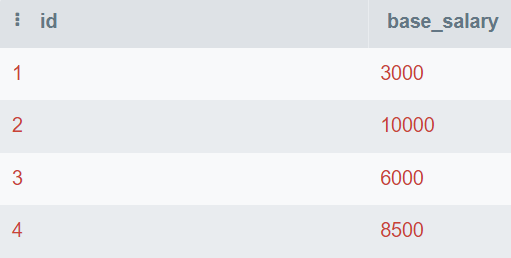Image by Author

In this query, you will find the unique values and order them from highest to lowest. Then, you will use `LIMIT 1` to display only the highest value. In the end, you will offset the value by 1 to display the second-highest number.

You can also change the `OFFSET` value to get nth highest salary.

``````SELECT DISTINCT base_salary AS "Second Highest Salary"
FROM employee
ORDER BY base_salary DESC
LIMIT 1
OFFSET 1;``````

The second highest base salary is 8,500.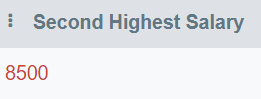### Find the duplicate emails

In this question, you will write a query to display all of the duplicate emails.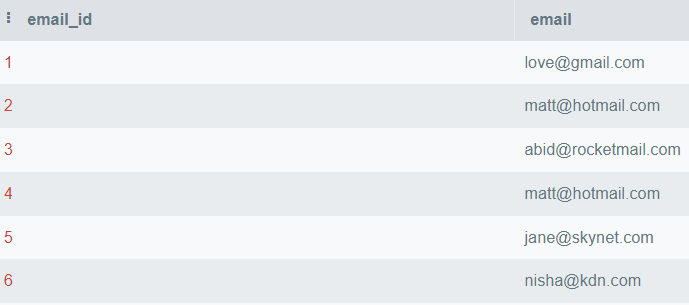Image by Author

In this query, you will display an `email` column and group the table by `email`. After that, we will use the `HAVING` clause to find emails that are mentioned more than once.

`HAVING` is used as a replacement for the `WHERE` statement in conjunction with aggregations.

``````SELECT email
FROM employee_email
GROUP BY email
HAVING COUNT(email) > 1;``````

Only “[email protected]” occurs more than once.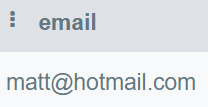Write maintainable SQL code to answer business questions by taking the Applying SQL to Real-World Problems course.

## FAANG Data Science Question

### Facebook Data Science Interview Question

#### Facebook composer, the posting tool, dropped from 3% posts per user last month to 2.5% posts per user today. How would you investigate what happened?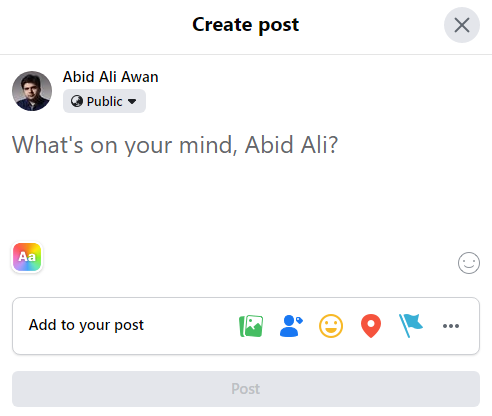Image by Author

The post from a month ago dropped from 3% to 2.5% today. Before you jump to a conclusion, you need to clarify the context around the problem.

• Is it a weekday today?
• Was a month ago from today a weekend?
• Are there special occasions, events, or seasonality?
• Is there a gradual downward trend or a one-time occurrence?

In the second part, you need to elaborate on what drove the decrease. Has the number of users increased, or the number of posts has decreased? After that, the interviewer will ask to initiate a discussion using one or both reasoning.

#### What do you think the distribution of time spent per day on Facebook looks like? What metrics would you use to describe that distribution?

In terms of the distribution of time spent per day on Facebook, one can assume that there might be two groups:

1. People who scroll through the feed fast without spending too much time.
2. Super users who spend large amounts of time on Facebook.

For the second part, you have to use statistical vocabulary to describe the distribution, such as:

1. Center: mean, median, and mode
2. Spread: standard deviation, interquartile range, and range
3. Shape: skewness, kurtosis, and uni or bimodal
4. Outliers

#### Given a dataset of test scores, write pandas code to return the cumulative percentage of students that received scores within the buckets of <50, <75, <90, <100.

In this question, you will write pandas code to first divide the score into various buckets and then calculate the percentage of the students getting the score in those brackets.

Input:

Our dataset has user_id, grade, and test_score columns.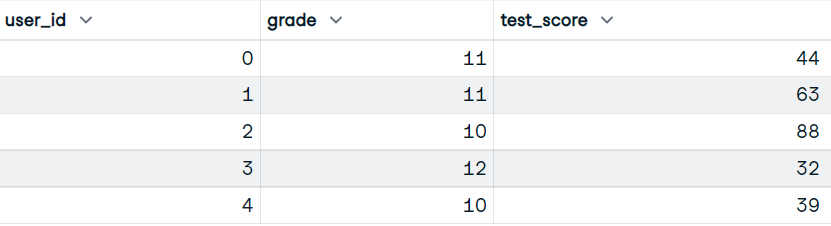Image by Author

Output:

You will be writing the function that will use grade and test_score columns. And display the dataframe with grades, bucket scores, and cumulative percentage of students getting bucket scores.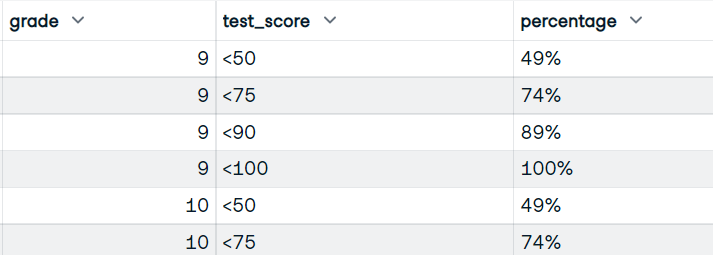Image by Author

1. You will use the `pandas.cut()` function to convert scores into bucket scores using bins and labels of buckets.
2. Calculate the size of each group (grade and test_score).
3. For calculating the percentage, we need numerator (cumulative sum) and denominator (sum of all values).
4. Converting the fraction value into a proper percentage by multiplying it by 100 and adding “%”.
5. Reset index and rename the column as "percentage".
``````def bucket_test_scores(df):
bins = [0, 50, 75, 90, 100]
labels = ["<50", "<75", "<90", "<100"]

# converting the scores into buckets
df["test_score"] = pd.cut(df["test_score"], bins, labels=labels, right=False)

# Calculate size of each group, by grade and test score

# Calculate numerator and denominator for percentage

# Calculate percentage, multiply by 100, and add %
percentage = (NUM / DEN).map(lambda x: f"{int(100*x):d}%")

# reset the index
percentage = percntage.reset_index(name="percentage")
return percentage

bucket_test_scores(df)``````

You got the perfect result with a bucket test score and the percentage.Learn how to clean data, calculate statistics, and create visualizations with Data Manipulation with pandas course.

### Amazon Data Science Interview Question

#### Explain confidence intervals

The confidence interval is a range of estimates for an unknown parameter that you expect to fall between a certain percentage of the time when you run the experiment again or similarly re-sample the population.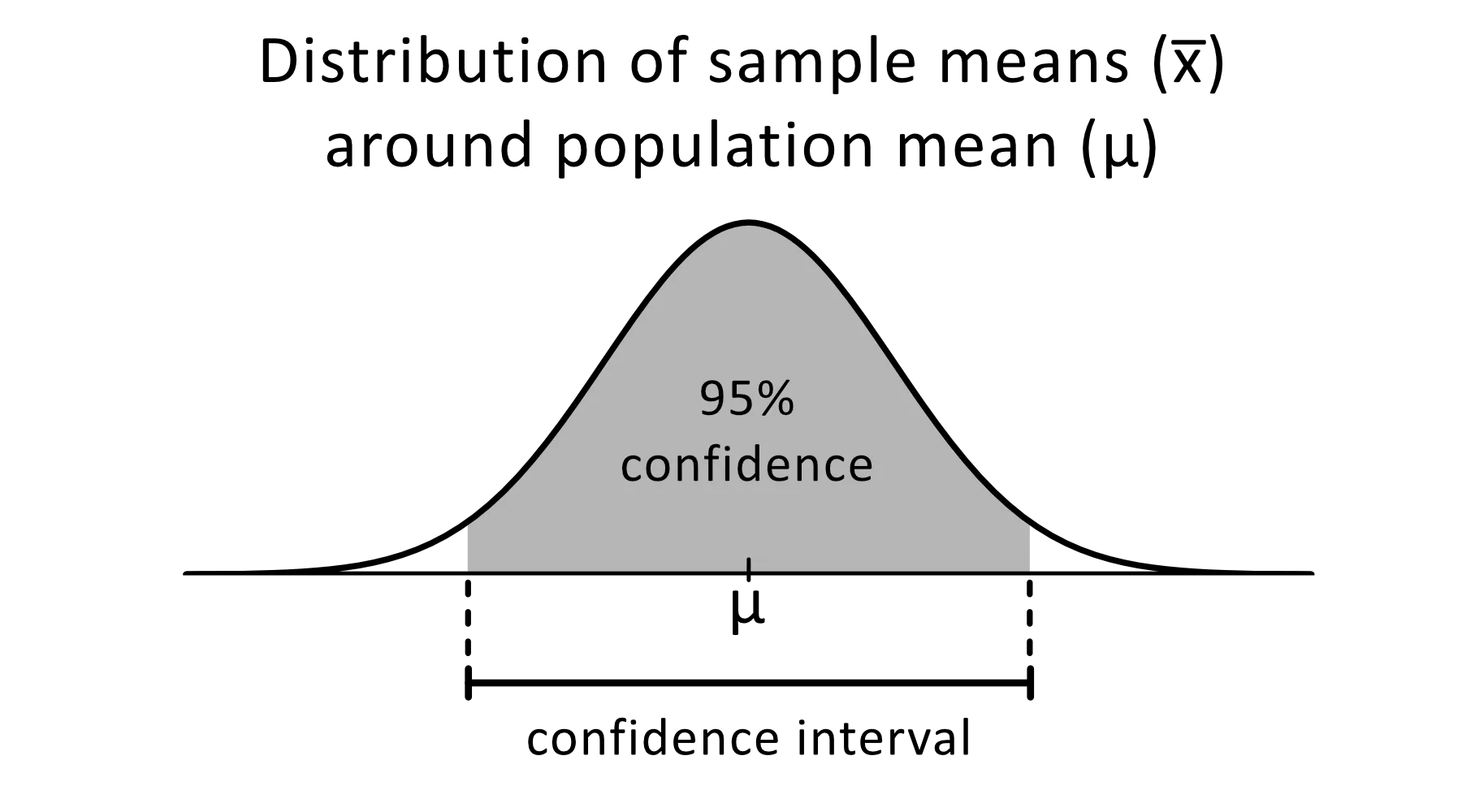Image from omnicalculator

The 95% confidence level is commonly used in statistical experiments, and it is the percentage of times you expect to reproduce an estimated parameter. The confidence intervals have an upper and lower bound that is set by the alpha value.

You can use confidence intervals for various statistical estimates, such as proportions, population means, differences between population means or proportions, and estimates of variation among groups.

Build the statistics foundation by completing our Statistical Thinking in Python (Part 1) course.

#### How do you manage an unbalanced dataset?

In the unbalanced dataset, the classes are distributed unequally. For example, in the fraud detection dataset, there are only 400 fraud cases compared to 300,000 non-fraud cases. The unbalanced data will make the model perform worse in detecting fraud.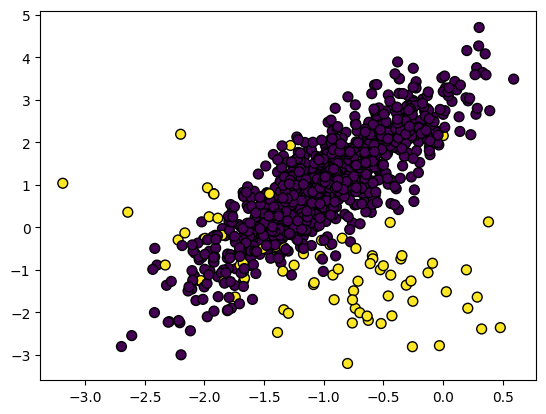Image by Author

To handle imbalanced data, you can use:

1. Undersampling
2. Oversampling
3. Creating synthetic data
4. Combination of under and over sampling

Undersampling

It resamples majority class features to make them equal to the minority class features.

In the fraud detection dataset, both classes will be equal to 400 samples. You can use `imblearn.under_sampling` to resample your dataset with ease.

``````from imblearn.under_sampling import RandomUnderSampler
RUS = RandomUnderSampler(random_state=1)
X_US, y_US = RUS.fit_resample(X_train, y_train)``````

Oversampling

It resamples minority class features to make them equal to the majority class features. Repetition or weightage repetition of the minority class features are some of the common methods used in balancing the data. In short, both classes will have 300K samples.

``````from imblearn.over_sampling import RandomOverSampler

ROS = RandomOverSampler(random_state=0)
X_OS, y_OS = ROS.fit_resample(X_train, y_train)``````

Creating synthetic data

The problem with repetition is that it does not provide extra information, which will result in the models' poor performance. To counter it, we can use SMOTE (Synthetic Minority Oversampling technique) to create synthetic data points.

``````from imblearn.over_sampling import SMOTE

SM = SMOTE(random_state=1)
X_OS, y_OS = SM.fit_resample(X_train, y_train)``````

Combination of under and over sampling

To improve model biases and performance, you can use a combination of over and under-sampling. We will use SMOTE for over-sampling and EEN (Edited Nearest Neighbours) for cleaning.

The `imblearn.combine` provides us with various functions that automatically perform both sampling functions.

``````from imblearn.combine import SMOTEENN

SMTN = SMOTEENN(random_state=0)
X_OUS, y_OUS = SMTN.fit_resample(X_train, y_train)``````

#### Write a query to return the total number of sales for each product for the month of March 2022.

As a data scientist, you will be writing a similar type of query to extract the data and perform data analysis. In this challenge, you will either use the `WHERE` clause with comparison signs or `WHERE` with `BETWEEN` clause to perform filtering.

Table: orders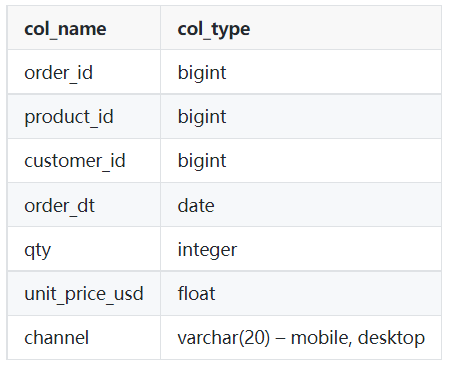Image by Author

Sample output: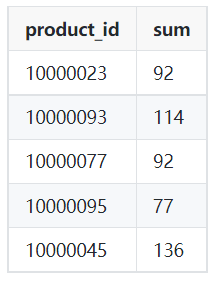Image by Author

1. You will display product id and sum of the quantity from the orders table.
2. Filter out the data from date ‘2022-03-01’  to '2022-04-01' using WHERE and AND clause. You can also use BETWEEN to perform a similar action.
3. Use a group by product_id to get the total number of sales for each product.
``````SELECT product_id,
SUM(qty)
FROM orders
WHERE order_dt >= '2022-03-01'
AND order_dt < '2022-04-01'
GROUP BY product_id;``````

### Google Data Science Interview Question

#### If the labels are known in a clustering project, how would you evaluate the performance of the model?

In unsupervised learning, finding the performance of the clustering project can be tricky. The criteria of good clustering are distinct groups with little similarity.

There is no accuracy metric in clustering models, so we will be using either similarity or distinctness between the groups to evaluate the model performance.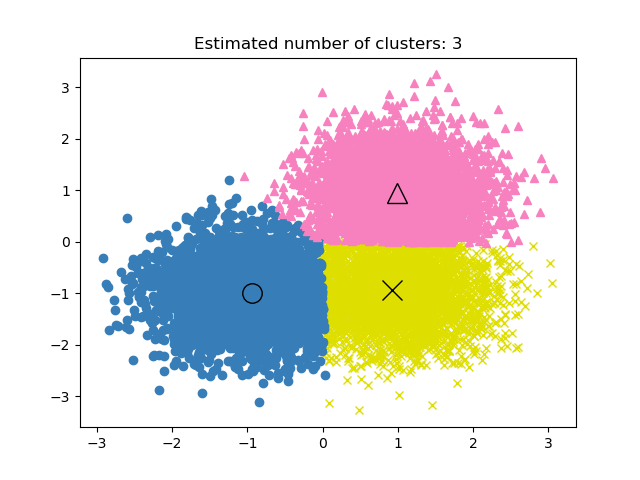Image from scikit-learn documentation

The three commonly used metrics are:

• Silhouette Score
• Calinski-Harabaz Index
• Davies-Bouldin Index

Silhouette Score

It is calculated using the mean intra-cluster distance and the mean nearest-cluster distance.

We can use scikit-learn to calculate the metric. The Silhouette Score is between -1 to 1, where higher scores mean lower similarity between groups and distinct clusters.

``````from sklearn import metrics

model = KMeans().fit(X)
labels = model.labels_

metrics.silhouette_score(X, labels)``````

Calinski-Harabaz Index

It calculates distinctiveness between groups using between-cluster dispersion and within-cluster dispersion. The metric has no bound, and just like Silhouette Score, a higher score means better model performance.

``metrics.calinski_harabasz_score(X, labels)``

Davies-Bouldin Index

It calculates the average similarity of each cluster with its most similar cluster. Unlike other metrics, a lower score means better model performance and better separation between clusters.

``metrics.davies_bouldin_score(X, labels)``

Learn how to apply hierarchical and k-means clustering by taking our Cluster Analysis in R course.

#### There are four people in an elevator and four floors in a building. What’s the probability that each person gets off on a different floor?Image by Author

We will use: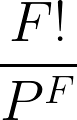• F = Number of floors
• P = Number of people

To solve this problem, we need to first find the total number of ways to get off the floors: 44 = 4x4x4x4 = 256 ways.

After that, calculate the number of ways each person can get off on a different floor: 4! = 24.

To calculate the probability that each person gets off on a different floor, we need to divide the number of ways each person gets off on a different floor with the total number of ways to get off the floors.

24/256 = 3/32

Learn strategies for answering tricky probability questions with R by taking our Probability Puzzles in R course.

#### Write a function to generate N samples from a normal distribution and plot the histogram.

To generate N samples from the normal distribution, you can either use Numpy `(np.random.randn(N))` or SciPy `(sp.stats.norm.rvs(size=N))`

To plot a histogram, you can either use Matplotlib or Seaborn.

The question is quite simple if you know the right tools.

1. You will generate random normal distribution samples using the Numpy randn function.
2. Plot histogram with KDE using Seaborn.
3. Plot histogram for 10K samples and return the Numpy array.
``````import numpy as np
import seaborn as sns

N = 10_000

def norm_dist_hist(N):
# Generating Random normal distribution samples
x = np.random.randn(N)
# Plotting histogram
sns.histplot(x, bins = 20, kde=True);
return x

X = norm_dist_hist(N)``````Learn how to create informative and attractive visualizations in seconds by completing Introduction to Data Visualization with Seaborn course.

##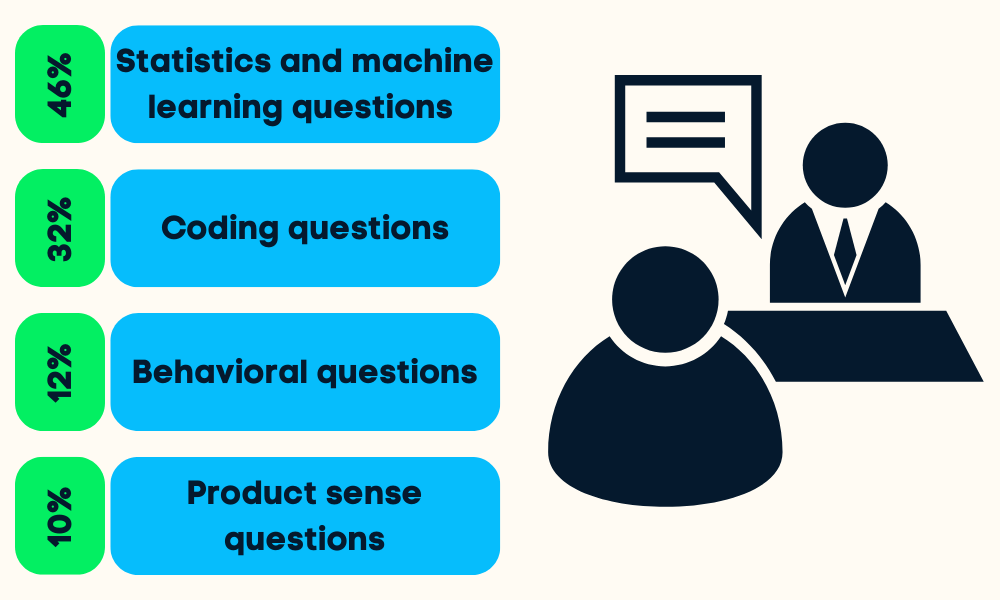Image by Author

The data science interviews are divided into four to five stages. You will be asked about statistical and machine learning, coding (Python, R, SQL), behavioral, product sense, and sometimes leadership questions.

You can prepare for all stages by:

1. Researching the company and job responsibilities: it will help you prioritize your effort in a certain field of data science.
3. Revising the data science fundamentals: probability, statistics, hypothesis testing, descriptive and bayesian statistics, and dimensionality reduction. Cheat sheets are the best way to learn the basics fast.
4. Practicing coding: take assessment tests, solve online code challenges, and review most asked coding questions.
5. Practicing on end-to-end projects: brush off your skills by data cleaning, manipulation, analysis, and visualization.
6. Reading the most common interview questions:  product sense, statistical, analytical, behavioral, and leadership questions.
7. Taking mock interview: practice an interview with a friend, improve your statical vocabulary, and become confident.

Read the Data Science Interview Preparation blog to learn what to expect and how to approach the interview.

## Data Science Interview FAQs

### What are the four major components of data science?.css-1dm0cr5-FAQEntry{-webkit-flex-shrink:0;-ms-flex-negative:0;flex-shrink:0;height:18px;padding-top:6px;-webkit-transform:rotate(0.5turn) translate(21%, -10%);-moz-transform:rotate(0.5turn) translate(21%, -10%);-ms-transform:rotate(0.5turn) translate(21%, -10%);transform:rotate(0.5turn) translate(21%, -10%);-webkit-transition:-webkit-transform 0.3s cubic-bezier(0.85, 0, 0.15, 1);transition:transform 0.3s cubic-bezier(0.85, 0, 0.15, 1);width:18px;}

The four Major components of data science are:

• Business understanding and data strategy.
• Data Preparation (Cleaning, Imputing, validating).
• Data Analysis and Modeling.
• Data Visualization and Operationalization.

### Are data science interviews hard?.css-qcen5k-FAQEntry{-webkit-flex-shrink:0;-ms-flex-negative:0;flex-shrink:0;height:18px;padding-top:6px;-webkit-transform:none;-moz-transform:none;-ms-transform:none;transform:none;-webkit-transition:-webkit-transform 0.3s cubic-bezier(0.85, 0, 0.15, 1);transition:transform 0.3s cubic-bezier(0.85, 0, 0.15, 1);width:18px;}

Yes. To pass a data science interview, you have to demonstrate proficiency in multiple areas such as statistics & probability, coding, data analysis, machine learning, product sense, and reporting.

### Is data science a stressful job?

It depends. Your whole team/company may rely on you to provide analysis and actionable information. In some cases, you have to wear multiple hats, such as data engineer, data analyst, machine learning engineer, MLOps engineer, data manager, and team lead. Some individuals find this exciting and challenging, while others find it stressful and overwhelming at times.

### Is one year enough for data science?

Maybe. It depends on your background. If you are working as a software engineer and want to switch, you can learn most of the things in a year. But, if you are starting from zero, it will be hard for you to become job-ready in a year. Start your journey as a Data Scientist with Python and learn all of the fundamentals in 6 months.

### Is data science math heavy?

Yes. You need to learn statistics, probability, math, data analysis, data visualization, and building and evaluating machine learning models.

### Is data science a good salary?

Yes. According to Glassdoor, the data scientist in the USA receives total pay of \$124,817 per year. The median salary of a Data Scientist globally is \$70,714 per year.

Data Science Courses

### .css-1531qan{-webkit-text-decoration:none;text-decoration:none;color:inherit;}Intermediate Python

Beginner
4 hr
947.9K
Level up your data science skills by creating visualizations using Matplotlib and manipulating DataFrames with pandas.
See Details

### Data Manipulation in SQL

Beginner
4 hr
172.9K
Master the complex SQL queries necessary to answer a wide variety of data science questions and prepare robust data sets for analysis in PostgreSQL.

### Python Data Science Toolbox (Part 1)

Beginner
3 hr
363.6K
Learn the art of writing your own functions in Python, as well as key concepts like scoping and error handling.
See More
Related

### Building Your Data Science Portfolio with DataCamp Workspace (Part 1)

Learn how to build a comprehensive data science portfolio by exploring examples different examples, mastering tips to make your work stand out, and utilizing the DataCamp Workspace effectively to showcase your results.9 min### [Radar Recap] Building an Enterprise Data Strategy that Puts People First

Cindi Howson and Valerie Logan discuss how data leaders can create a data strategy that puts their people at the center.### [Radar Recap] Unleashing the Power of Data Teams in 2023

Vijay Yadav and Vanessa Gonzalez will outline the keys to building high-impact data teams in 2023.### The Past, Present, and Future, of the Data Science Notebook

Jodie Burchell discusses notebooks and the challenges facing data science today.### Building a Safer Internet with Data Science

Learn the key drivers of a data strategy that helps ensure online safety and consumer protection with Richard Davis, the Chief Data Officer at Ofcom, the UK’s government-approved regulatory and competition authority.

### Conda Cheat Sheet

In this cheat sheet, learn all about the basics of working with Conda. From managing and installing packages, to working with channels & environments, learn the fundamentals of the conda package management tool suite.
See MoreSee More